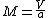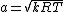# compressible flow

(redirected from Compressible fluid)

## Compressible flow

Flow in which density changes are significant. Pressure changes normally occur throughout a fluid flow, and these pressure changes, in general, induce a change in the fluid density. In a compressible flow, the density changes that result from these pressure changes have a significant influence on the flow. The changes in the flow that result from the density changes are often termed compressibility effects. All fluids are compressible. However, compressibility effects are more frequently encountered in gas flows than in liquid flows.

An important dimensionless parameter in compressible flows is the Mach number, M. This is defined by Eq. (1),

(1)where a is the speed of sound and V is the velocity of the flow. For a gas, the speed of sound is given by Eq. (2),
(2)where R is the gas constant, k = cp/cv, cp and cv being the specific heats at constant pressure and constant volume respectively, and T is the temperature. If M < 0.3 in a flow, the density changes in the flow will usually be negligible; that is, the flow can be treated as incompressible. Compressible flows are, therefore, as a rough guide, associated with Mach numbers greater than 0.3.

When M < 1, the flow is said to be subsonic; when M = 1, the flow is said to be sonic; when M varies from slightly below 1 to slightly above 1, the flow is said to be transonic; and if M > 1, the flow is said to be supersonic. When the Mach number is very high, this usually being taken to mean M > 5, the flow is said to be hypersonic.

Compressible flows can have features that do not occur in low-speed flows. For example, shock waves and expansion waves can occur in supersonic flows. Another important phenomenon that can occur due to compressibility is choking, where the mass flow rate through a duct system may be limited as a result of the Mach number being equal to 1 at some point in the flow. See Choked flow, Shock wave, Sonic boom

Another effect of compressibility is associated with the acceleration of a gas flow through a duct. In incompressible flow, an increase in velocity is associated with a decrease in the cross-sectional area of the duct, this in fact being true as long as M < 1. However, when M > 1, that is, when the flow is supersonic, the opposite is true; that is, an increase in the velocity is associated with an increase in the cross-sectional area. Therefore, in order to accelerate a gas flow from subsonic to supersonic velocities in a duct, it is necessary first to decrease the area and then, once the Mach number has reached 1, to increase the area, that is, to use a so-called convergent-divergent nozzle. An example is the nozzle fitted to a rocket engine. See Fluid flow, Mach number, Supersonic flow

McGraw-Hill Concise Encyclopedia of Physics. © 2002 by The McGraw-Hill Companies, Inc.

## compressible flow

[kəm′pres·ə·bəl ′flō]
(fluid mechanics)
Flow in which the fluid density varies.
McGraw-Hill Dictionary of Scientific & Technical Terms, 6E, Copyright © 2003 by The McGraw-Hill Companies, Inc.

## compressible flow

The flow of fluid in which its density varies. Compressible flow is encountered in supersonic flight.
An Illustrated Dictionary of Aviation Copyright © 2005 by The McGraw-Hill Companies, Inc. All rights reserved
References in periodicals archive ?
The Ukrainian authors analyze classical acoustic wave problems with an ideal compressible fluid model of media that takes into account the processes of generation, sound propagation, and interaction with obstacles.
When a compressible fluid experiences rapid heating, the fluid will expand.
In order to decrease complicated solving parameters in using compressible fluid method, geometry of every fuel assembly could be turned into a single heated channel (SHC).
Continuity equation for a compressible fluid is as follows:
To analyze the dynamic mechanical properties of the egg, Teixeira and Awruch  used a numerical simulation of the fluid-solid coupling system by means of the finite element method, conducted a numerical analysis of that system in Arbitrary Lagrangian-Eulerian (ALE) equations using the Taylor-Galerkin method, and verified their correctness with two numerical examples, that is, a compressible fluid and a slightly compressible fluid.
Biot and Willis  studied the methods of measurement to determine the elastic coefficients based on the theory of the deformation of a porous elastic solid containing a compressible fluid. They also discussed the physical interpretation of the coefficients in various alternate forms.
Zien, "On the inviscid stability of the laminar mixing of two parallel streams of a compressible fluid," Journal of Fluid Mechanics, vol.
To study the two-phase fluid compressibility, oil and water generally is known as micro-compressible fluid, and gas is known as a compressible fluid. Under isothermal conditions, Fluid compression factor [C.sub.f] can be given by,
[8.] Imrie, B.W., "Compressible Fluid Flow" London, Butterworths
Long-axis middle suprapatellar view (a), long-axis medial suprapatellar view (b), short-axis medial suprapatellar view (c), and compressible fluid collection in short-axis suprapatellar view (d).
The numerical investigation was carried out by full three-dimensional steady state compressible fluid CFD-simulation using Ansys Fluent, the employed model utilized the standard k-[epsilon] turbulence model to solve the flow equations.

Site: Follow: Share:
Open / Close